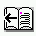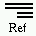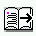DOC HOME SITE MAP MAN PAGES GNU INFO SEARCH PRINT BOOK

### Berkeley DB Reference Guide:Access Methods### Disk space requirements

It is possible to estimate the total database size based on the size of the data. The following calculations are an estimate of how many bytes you will need to hold a set of data and then how many pages it will take to actually store it on disk.

Space freed by deleting key/data pairs from a Btree or Hash database is never returned to the filesystem, although it is reused where possible. This means that the Btree and Hash databases are grow-only. If enough keys are deleted from a database that shrinking the underlying file is desirable, you should create a new database and copy the records from the old one into it.

These are rough estimates at best. For example, they do not take into account overflow records, filesystem metadata information, large sets of duplicate data items (where the key is only stored once), or real-life situations where the sizes of key and data items are wildly variable, and the page-fill factor changes over time.

### Btree

The formulas for the Btree access method are as follows:

```useful-bytes-per-page = (page-size - page-overhead) * page-fill-factor

bytes-of-data = n-records *

n-pages-of-data = bytes-of-data / useful-bytes-per-page

total-bytes-on-disk = n-pages-of-data * page-size
```

The useful-bytes-per-page is a measure of the bytes on each page that will actually hold the application data. It is computed as the total number of bytes on the page that are available to hold application data, corrected by the percentage of the page that is likely to contain data. The reason for this correction is that the percentage of a page that contains application data can vary from close to 50% after a page split to almost 100% if the entries in the database were inserted in sorted order. Obviously, the page-fill-factor can drastically alter the amount of disk space required to hold any particular data set. The page-fill factor of any existing database can be displayed using the db_stat utility.

The page-overhead for Btree databases is 26 bytes. As an example, using an 8K page size, with an 85% page-fill factor, there are 6941 bytes of useful space on each page:

`6941 = (8192 - 26) * .85`

The total bytes-of-data is an easy calculation: It is the number of key or data items plus the overhead required to store each item on a page. The overhead to store a key or data item on a Btree page is 5 bytes. So, it would take 1560000000 bytes, or roughly 1.34GB of total data to store 60,000,000 key/data pairs, assuming each key or data item was 8 bytes long:

`1560000000 = 60000000 * ((8 + 5) * 2)`

The total pages of data, n-pages-of-data, is the bytes-of-data divided by the useful-bytes-per-page. In the example, there are 224751 pages of data.

`224751 = 1560000000 / 6941`

The total bytes of disk space for the database is n-pages-of-data multiplied by the page-size. In the example, the result is 1841160192 bytes, or roughly 1.71GB.

`1841160192 = 224751 * 8192`

### Hash

The formulas for the Hash access method are as follows:

```useful-bytes-per-page = (page-size - page-overhead)

bytes-of-data = n-records *

n-pages-of-data = bytes-of-data / useful-bytes-per-page

total-bytes-on-disk = n-pages-of-data * page-size
```

The useful-bytes-per-page is a measure of the bytes on each page that will actually hold the application data. It is computed as the total number of bytes on the page that are available to hold application data. If the application has explicitly set a page-fill factor, pages will not necessarily be kept full. For databases with a preset fill factor, see the calculation below. The page-overhead for Hash databases is 26 bytes and the page-overhead-for-two-entries is 6 bytes.

As an example, using an 8K page size, there are 8166 bytes of useful space on each page:

`8166 = (8192 - 26)`

The total bytes-of-data is an easy calculation: it is the number of key/data pairs plus the overhead required to store each pair on a page. In this case that's 6 bytes per pair. So, assuming 60,000,000 key/data pairs, each of which is 8 bytes long, there are 1320000000 bytes, or roughly 1.23GB of total data:

`1320000000 = 60000000 * (16 + 6)`

The total pages of data, n-pages-of-data, is the bytes-of-data divided by the useful-bytes-per-page. In this example, there are 161646 pages of data.

`161646 = 1320000000 / 8166`

The total bytes of disk space for the database is n-pages-of-data multiplied by the page-size. In the example, the result is 1324204032 bytes, or roughly 1.23GB.

`1324204032 = 161646 * 8192`

Now, let's assume that the application specified a fill factor explicitly. The fill factor indicates the target number of items to place on a single page (a fill factor might reduce the utilization of each page, but it can be useful in avoiding splits and preventing buckets from becoming too large). Using our estimates above, each item is 22 bytes (16 + 6), and there are 8166 useful bytes on a page (8192 - 26). That means that, on average, you can fit 371 pairs per page.

`371 = 8166 / 22`

However, let's assume that the application designer knows that although most items are 8 bytes, they can sometimes be as large as 10, and it's very important to avoid overflowing buckets and splitting. Then, the application might specify a fill factor of 314.

`314 = 8166 / 26`

With a fill factor of 314, then the formula for computing database size is

`n-pages-of-data = npairs / pairs-per-page`

or 191082.

`191082 = 60000000 / 314`

At 191082 pages, the total database size would be 1565343744, or 1.46GB.

`1565343744 = 191082 * 8192`

There are a few additional caveats with respect to Hash databases. This discussion assumes that the hash function does a good job of evenly distributing keys among hash buckets. If the function does not do this, you may find your table growing significantly larger than you expected. Secondly, in order to provide support for Hash databases coexisting with other databases in a single file, pages within a Hash database are allocated in power-of-two chunks. That means that a Hash database with 65 buckets will take up as much space as a Hash database with 128 buckets; each time the Hash database grows beyond its current power-of-two number of buckets, it allocates space for the next power-of-two buckets. This space may be sparsely allocated in the file system, but the files will appear to be their full size. Finally, because of this need for contiguous allocation, overflow pages and duplicate pages can be allocated only at specific points in the file, and this too can lead to sparse hash tables.